# Tits system

(diff) ← Older revision | Latest revision (diff) | Newer revision → (diff)

A collection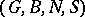, whereis a group,andare subgroups andis a subset of, satisfying the following conditions: 1) the set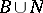generates the group; 2)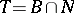is a normal subgroup of; 3) the setgenerates the groupand consists of elements of order 2; 4)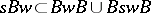for all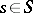,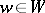; and 5)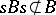for. The group, called the Weyl group of the Tits system, is a Coxeter group with respect to the system of generators. The correspondence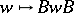is a bijection fromonto the set of double cosets ofin.

Examples. a), whereis any field,is the subgroup of upper triangular matrices,is the subgroup of monomial matrices (so thatis the subgroup of diagonal matrices and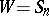, cf. Monomial matrix), andis the set of transpositions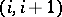, where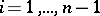.

b) More generally, letbe a connected reductive algebraic group over, letbe a maximal torus indiagonalizable over, letbe its normalizer, let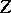be its centralizer, letbe the root system ofrelative to, let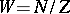be its Weyl group, and letbe the set of reflections corresponding to the simple roots. Moreover, letbe the unipotent subgroup ofgenerated by the root subgroups corresponding to the positive roots, and let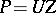. Then the quadruple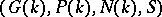is a Tits system.

c) Let, where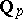is the field of-adic numbers, letbe the subgroup consisting of matrices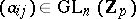(where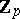is the ring of-adic integers), such thatfor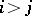, and letbe the subgroup of monomial matrices. Then there exists a subset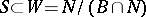such that the quadrupleis a Tits system. Here the groupis an infinite Coxeter group of type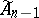. Analogously one can define Tits systems with Weyl groups of affine type corresponding to other connected reductive groups over local fields.

Under certain conditions one can assert that a groupadmitting a Tits system is simple. For example, the following conditions are sufficient for this:)is a solvable group, and is not contained in any proper normal subgroup of;)is equal to its own commutator subgroup;) the Coxeter groupis indecomposable; or) the groupdoes not contain any non-trivial normal subgroup of. In this way one can prove the simplicity of the Chevalley groups, in particular of the finite ones (cf. Chevalley group).

How to Cite This Entry:
Tits system. Encyclopedia of Mathematics. URL: http://encyclopediaofmath.org/index.php?title=Tits_system&oldid=11480
This article was adapted from an original article by E.B. Vinberg (originator), which appeared in Encyclopedia of Mathematics - ISBN 1402006098. See original article